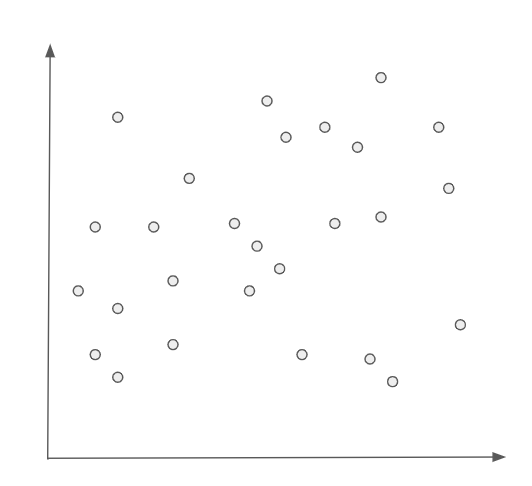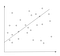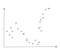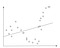# Predicting Unpredictable Probabilities

Deep Neural Networks and Why They Rule the World (Mostly)

A significant portion of machine learning approaches are linear in nature — i.e. they take a look at training data observations and try to find the slope and intercept of the line that best fits that data. For example, let’s assume we have a training data set that has a shape something like this:It’s like an ink-blot test, but for data nerds. What shapes to you see?

While there are some outlying observations, the general trend for this data is as the x-axis moves to the right, the y-axis goes up. Therefore, a linear model is an appropriate approach for inference. The prediction visualized might look something like this:This is art, not science. Zero points awarded for pointing out my line is incorrect.

This is all well and good, so long as your data have a relatively straightforward distribution like this. However, in the real world, for really complex data and predictions, the data are rarely that straightforward. Take, for example, the scenario where your data look like this:OK, this one is definitely a bird. Or a seahorse, maybe?

You can still use linear models to find the “best fit” from a mathematical perspective. It might look something like this:Again, no points for making the line more accurate. Stop judging me! :)

In all likelihood, however, this model is going to perform very poorly in the real world. When you run into these kinds of data, what you really need are machine learning models that can learn more complex functions, and at the end, give you something that looks more like this from a prediction standpoint:

There are two primary ways I know of to approach this: Decision Trees, and Neural Networks. For really, really complicated problems like images and language, Neural Networks currently rule the world, and for standard tabular data, . So how do they work and what makes them so powerful?

At a basic level, Neural Networks are very similar to our old friend the Perceptron. They start by working through the data and generating a linear separator.

At the point where the error passes a certain threshold, the Neural Network will stop, mark its spot, and change direction in the direction of the error, once again generating a linear separator, but this time starting at that point. It would visualize something like this:

This process repeats, and can repeat as many times as needed to achieve a predictive model with an arbitrarily good fit. A simple example on our current image might look something like this: